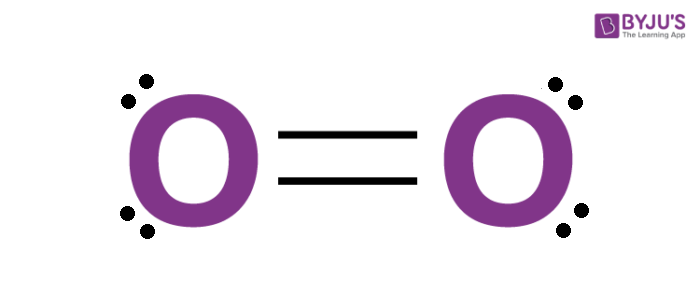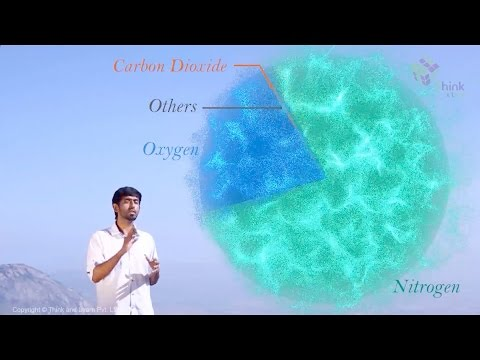Checkout JEE MAINS 2022 Question Paper Analysis : Checkout JEE MAINS 2022 Question Paper Analysis :

# Preparation, Properties & Uses Of Dioxygen

## What is Dioxygen?

Dioxygen plays a vital part in the existence of life. It is a highly reactive non-metal and it is a member of the chalcogen group on the modern periodic table. Oxygen is diatomic molecule and hence two atoms of the elements combine to form dioxygen. It is the third most abundant element in the universe.

Around 21% of the earth’s atmosphere consists of this gas in its free state. Chemical formula of oxygen is

$$\begin{array}{l}O_2\end{array}$$
. It is the most abundant element found in the earth’s crust.## Recommended Videos## Laboratory Preparation of Oxygen

In the laboratory, oxygen can be prepared in multiple ways:

• Hydrogen peroxide in the presence of finely divided metals and manganese dioxide decomposes to give water and dioxygen.

$$\begin{array}{l}2H_2O_2 ~(aq) \rightarrow~ 2H_2O~ (l)~ +~ O_2~ (g)\end{array}$$

• Oxides of few metals decompose in the presence of heat to give

$$\begin{array}{l}O_2\end{array}$$
.

$$\begin{array}{l}2HgO~ (s)~ \rightarrow~ 2Hg~ (l)~ +~ O_2~ (g)\end{array}$$

$$\begin{array}{l}2PbO_2 ~(s) ~\rightarrow~ 2PbO~ (s)~ +~ O_2~ (g)\end{array}$$

• Salts of chlorates, permanganates and nitrates decompose on heating to give

$$\begin{array}{l}O_2\end{array}$$
.

$$\begin{array}{l}2KClO_3~ \rightarrow~ 2KCl~ +~3O_2\end{array}$$

Industrial preparation of

$$\begin{array}{l}O_2\end{array}$$
:

$$\begin{array}{l}O_2\end{array}$$
is obtained on a large scale from air. Carbon dioxide and water is removed from air and the remaining gases are eliminated by fractional distillation and liquefaction which releases dinitrogen and
$$\begin{array}{l}O_2\end{array}$$
.

## Properties of Dioxygen

• It is a colourless, odourless diatomic gas and paramagnetic in nature.
Oxygen is highly reactive non-metal.
• This diatomic gas is a strong oxidizing gas.

$$\begin{array}{l}2SO_2~ +~ O_2~\rightarrow~ 2SO_3\end{array}$$

• It is second most electronegative element after fluorine.
• Dioxygen reacts with metals, non-metals to give oxides of the respective element.
$$\begin{array}{l}2Ca~ +~ O_2 ~\rightarrow~ 2CaO\end{array}$$

## Uses of Dioxygen

Oxygen is used in many applications. Oxygen is used:

• As an oxygen supplement in medicine.
• For respiration purpose.
• In the industries for the smelting of iron ore to form steel.
• In metal cutting and welding.
• As an oxidizer in water treatment and rocket fuel.

For a detailed discussion on preparation, properties and uses of dioxygen please visit BYJU’S.

Test your knowledge on Dioxygen Preparation Properties Uses